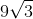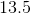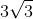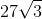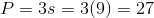# SSAT Upper Level Math : How to find the perimeter of an equilateral triangle

## Example Questions

### Example Question #81 : Properties Of Triangles

The length of a side in a equilateral triangle is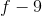. Find the perimeter of this triangle.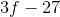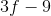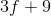Explanation:

Since an equilateral triangle has side lengths that are all the same, multiply the length of one side byto find the perimeter.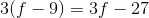### Example Question #82 : Properties Of Triangles

The length of one side of an equilateral triangle is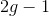. What is the perimeter for this triangle?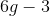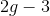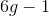Explanation:

Since an equilateral triangle has sides that are all equal, multiply the length of one side byto find the perimeter.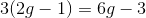### Example Question #83 : Properties Of Triangles

The length of one side of an equilateral triangle is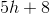. What is the perimeter for this triangle?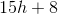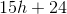Explanation:

Since an equilateral triangle has sides that are all equal, multiply the length of one side byto find the perimeter.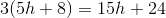### Example Question #84 : Properties Of Triangles

The length of one side of an equilateral triangle is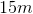. In meters, what is the perimeter of this triangle?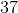Explanation:

Since an equilateral triangle has equal side lengths, multiply the length of one side byto find the perimeter.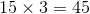### Example Question #85 : Properties Of Triangles

The length of one side of an equilateral triangle is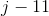. What is the perimeter fo this triangle?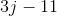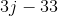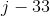Explanation:

Since an equilateral triangle has sides of equal length, multiply the length of one side byto find the perimeter.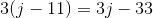### Example Question #86 : Properties Of Triangles

A side of an equilateral triangle has a length of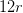. What is the perimeter of the triangle?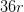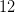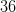Explanation:

Since an equilateral triangle has equal side lengths, multiply one side length by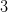to find the perimeter.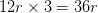### Example Question #87 : Properties Of Triangles

The length of one side of an equilateral triangle is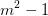. What is the perimeter of the triangle?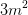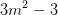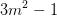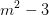Explanation:

Since the side lengths of a triangle are equal, multiply the length of one side byto find the perimeter.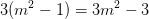### Example Question #88 : Properties Of Triangles

What is the perimeter of an equilateral triangle with a side of?